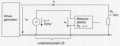# Determine inteference voltage with an inductance

#### Jiwoo

Joined Feb 6, 2023
19
Hi, I have the following circuit given:The task statement says "The strip conductor LB that is common to both circuits has an inductance of 100mH. The resistance of the common trace portion is negligibly small. The amplitude of u2 is 10V and f=1MHz. Due to the galvanic coupling via the trace component LB, there is an interference voltage at the measuring amplifier. Determine the interference voltage."

I am not sure, where the interference voltage is applied and how to determine it. I think the interference voltage is uv nad my equivalent circuit looks like this:But this would mean that uv equals u2 which I think is wrong. Do I need to consider R2 as well? How would your equivalent circuit look like? I would be very thankful if you help me solving this.

#### ericgibbs

Joined Jan 29, 2010
18,021
hi @Jiwoo
I agree , the circuit is a little confusing.
Can you confirm that the . The strip conductor LB that is common to both circuits has an inductance of 100mH

This inductance value seems very high for a copper track.?

E
Clip:
The inductance of a ~1 in. trace is typically quoted as being 5 to 10 nH. For the optimized trace I've designed with this model, the total inductance for a length of 1 in. is 7.4168 nH, which is within the range normally measured for small PCB traces.

Last edited:
@ericgibbs indeed i made a stupid mistakeIt is 100nH you are right
•